Plots

Plots

 We explain here how to find TANLINE, SLOPE, EXTREM, AREA, SHADE and how to use ZOOM of a FUNCTION PLOT. HP48/49 has a powerfull plot application, but it is redundant explain here all kind of plots because it is included in the Users Guide. All pictures show the plot of y=sin(x) with angle mode set for radians (RAD). You need redraw the plot every time you execute a new command. Legend: BLUE- titles [RED]- softkeys or menu keys.

The Plots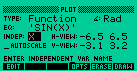Plot Application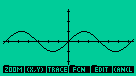Plot of Y=sin(x) 1) (X,Y) Press (X,Y) move the cursor up or down, left or right to see its position on the screen.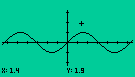2) Trace Press [TRACE] and (X,Y), move the cursor left or right to see its position on the line.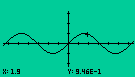3) ZOOM BOX - shows the zoom to a box. First move the cursor to a point of the screen so press [ZOOM]  [BOXZ] and draw a box.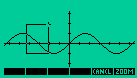Now press [ZOOM] other timeto see the result. Use [ZDFLT] to set the plot to the default zoom.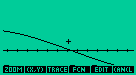4) ZOOM IN Press [ZOOM] [ZIN] to see the plot bigger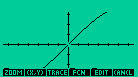5) ZOOM OUT Press [ZOOM][ZOUT] see the plot smaller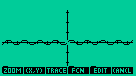6) ZOOM FACTOR Zoom factor permits you adjust the zoom magnifying factor. Press [ZOOM][ZDFLT] to set the zoom factor.7) ROOT To find a root of an equation set the cursor at the point closest to the root you wish find. And so press [ FCN ] [ROOT]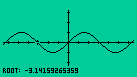8) INTERSECTION To find the intersection of two equations go with the cursor to a point closest to the intersection. And so press [ FCN ] [ISECT]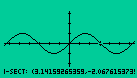9) AREA We need define two points on the X axe to calculate the area. First move the cursor to the first point and press [ FCN ] [AREA] and then move the cursor to the second point and press [AREA]10) SHADOW We need define two points on the X axe to shadow a plot. First move the cursor to the first point and press [ FCN ] [SHADE] and then move the cursor to the second point and press [SHADE]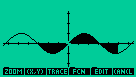11) EXTREM To find the extrem point of a plot function, plot the function and press [ FCN ] [EXTRM]12) SLOPE To find the slope at a given point of a curve, first move the cursor to a point on the curve and press [ FCN ] [SLOPE]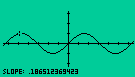13) TANLINE To find a stright tanline that passes for a given point of a plot, move the cursor to a point on the curve and press [ FCN ] [TANL]14) F' This function plots a second plot, i.e. the plot of the derivative of the original plot. Draw the plot of a function and press [ FCN ] [F'] to execute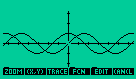15) F(X) This function calculates the value of y=f(x). Move the cursor to a point in the screen and press [ FCN ] [F(x)] to see the value of the function, in the point.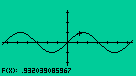16) Multiples Plots Enter a list of expressions delimited by an apostrofe in the EQ field to plot several functions. Example: {'sin(x)' 'x^2' '3' }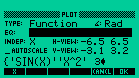HP48 will draw the plots of all functions.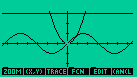17) LABEL Pressing [EDIT] [LABEL] you can see the coordinates in the screen.18) [EDIT] Pressing [EDIT] you can draw lines,circles and boxes, erase lines and paint.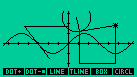We are litle creatures. In size the midway, between atoms and stars. Carl Sagan